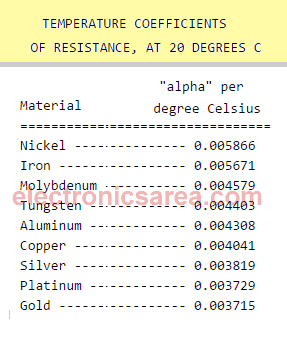Temperature effects on resistance

Resistance Variation with Temperature

The value of the resistance of a material varies with temperature change. For this reason, the circuit or system containing these elements should work in controled environments where the temperature is normal and constant.

If the temperature is not the right one, the resistance value will be different. The new resistance value, for a new temperature, is obtained using the formula: RTF = Rto x [1 + α (tf – to)] , where

• RTF = final temperature resistance in ohms
• Rto = initial temperature to resistance in ohms
• α = temperature coefficient per degree celsius (see table below)
• Tf = final temperature in °C
• To = initial temperature in °C

Table of temperature coefficients of resistance, at 20°CExample #1: We have a copper conductor wire with resistance = 20 ohms, at a temperature of 10 °C. What will be the new resistance value, if it rises to 70°C?

Applying the formula: RTF = Rto x [1 + α (tf – to)], with the following values:

– Rto = 20 ohms
– α = 0.004041 (copper)
– Tf = 70 ° C
– To = 10 ° C

We get: RTF = 20 [1 + 0.004041 (70-10)] = 24,85 ohms. (the resistance value increased 4.85 ohms of its original value)

Example #2: We have a aluminium conductor wire with resistance = 30 ohms, at a temperature of 25 °C. What will be the new resistance value, if it falls to 15°C?

Applying the formula: RTF = Rto x [1 + α (tf – to)], with the following values:

– Rto = 30 ohms
– Α = 0.003729 (aluminium)
– Tf = 15°C
– To = 25°C

We get: RTF = 30 [1 + 0.003729 (15-25)] = 28,88 ohms. (the resistance value decreased 1.12 ohms of its original value).

• 2
•
•
•
•
•
•
•
•
2
Shares
•
2
Shares
• 2
•
•
•
•
•
•
•